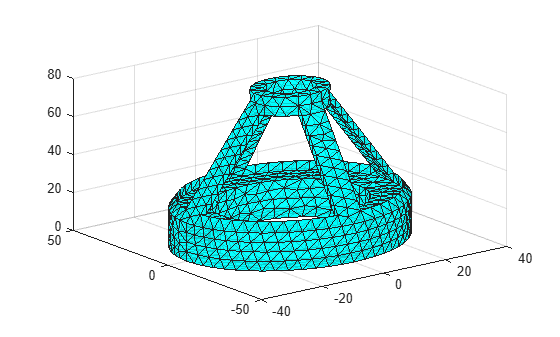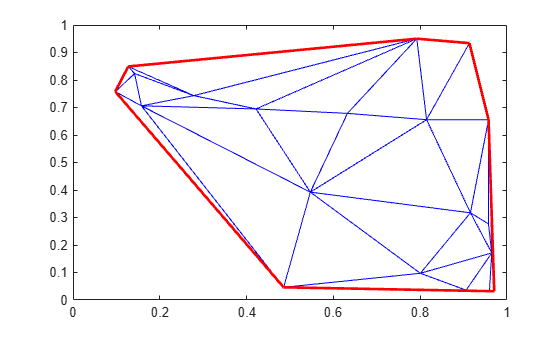# freeBoundary

(Not recommended) Free boundary facets

`freeBoundary(TriRep)` is not recommended. Use `freeBoundary(triangulation)` instead.

`TriRep` is not recommended. Use `triangulation` instead.

## Syntax

``FF = freeBoundary(TR)``
``[FF,XF] = freeBoundary(TR)``

## Description

example

````FF = freeBoundary(TR)` returns a matrix `FF` that represents the free boundary facets of the triangulation. A facet is on the free boundary if it is referenced by only one simplex.```

example

````[FF,XF] = freeBoundary(TR)` also returns a matrix of vertex coordinates for the free boundary facets.```

## Examples

collapse all

Use `TriRep` to compute the boundary triangulation of an imported triangulation.

```load tetmesh; trep = TriRep(tet,X);```

Compute the boundary triangulation.

`[tri,xf] = freeBoundary(trep);`

Plot the boundary triangulation.

```trisurf(tri,xf(:,1),xf(:,2),xf(:,3), ... 'FaceColor','cyan','FaceAlpha',0.8)```Perform a direct query of a 2-D triangulation created with `DelaunayTri`.

Create a Delaunay triangulation.

```x = rand(20,1); y = rand(20,1); dt = DelaunayTri(x,y);```

Compute the free boundary of the triangulation, and then plot the triangulation with the free boundary edges in red.

```fe = freeBoundary(dt)'; triplot(dt) hold on plot(x(fe),y(fe),'-r','LineWidth',2) hold off```In this instance the free edges correspond to the convex hull of (x,y).

## Input Arguments

collapse all

Triangulation representation, specified as a `TriRep` or `DelaunayTri` object.

## Output Arguments

collapse all

Free boundary facets, returned as a matrix. `FF` is of size `m`-by-`n`, where `m` is the number of boundary facets and `n` is the number of vertices per facet. The vertices of the facets index into the array of points representing the vertex coordinates `TR.X`. The array `FF` could be empty as in the case of a triangular mesh representing the surface of a sphere.

Vertex coordinates of free boundary facets, returned as a matrix. `XF` is of size `m`-by-`ndim`, where `m` is the number of free facets, and `ndim` is the dimension of the space where the triangulation resides.

collapse all

### Simplex

A simplex is a triangle/tetrahedron or higher-dimensional equivalent.

### Facet

A facet is an edge of a triangle or a face of a tetrahedron.